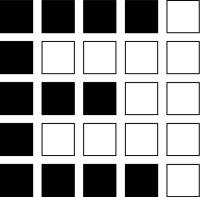# 17.4 Summary

The Hopfield model is an abstract model of memory retrieval. After a cue with a partial overlap with one of the stored memory patterns is presented, the memory item is retrieved. Because the Hopfield model has symmetric synaptic connections, memory retrieval can be visualized as downhill movement in an energy landscape. An alternative view is that of memories forming attractors of the collective network dynamics. While the energy picture does not carry over to networks with asymmetric interactions, the attractor picture remains applicable even for biologically more plausible network models with spiking neurons.

Attractor networks where each neuron participates in several memory patterns can be seen as a realization of Hebb’s idea of neuronal assemblies. At the current state of research, it remains unclear whether increased spiking activity observed in cortex during delayed matching-to-sample tasks has a relation to attractor dynamics. However, the ideas of Hebb and Hopfield have definitely influenced the thinking of many researchers.

# Literature

Precursors of the Hopfield model are the networks models of Willshaw et al. (549); Kohonen (270); Anderson (26); Little (304). The model of associative memory of Willshaw et al. (549) was designed for associations between a binary input pattern $\xi^{\mu,A}$ and a binary output vector $\xi^{\mu,B}$ where $\xi_{i}^{\mu,A/B}\in\{0,1\}$ and interactions weights $w_{ij}$ are taken $\propto\xi_{i}^{\mu,B}\,\xi_{j}^{\mu,A}$ where $j$ is a component of the input and $i$ a component of the desired output pattern. A recurrent network can be constructed, if the dimensionality of inputs and outputs match and the output from step $n$ is used as input to step $n+1$.

Intrinsically recurrent models of memory were studied with linear neurons by (270; 26) and with stochastic binary units by (304). The latter showed that, under some assumptions, persistent states, that can be identified with potential memory states, can exist in such a network.

Hopfield’s paper in 1982 has influenced a whole generation of physicists and is probably the most widely cited paper in computational neuroscience. It initiated a wave of studies of storage capacity and retrieval properties in variants of the Hopfield model using the tools of statistical physics (18; 22) including extensions to low-activity patterns (19; 522), sparsely connected networks (123) and temporal sequences (488; 217). The energy function in (226) requires symmetric interactions, but the dynamics can also be analyzed directly on the level of overlaps. The book of Hertz et al. (215) presents an authoritative overview of these and related topics in the field of associative memory networks.

The transition from abstract memory networks to spiking network models has been started after 1990 (23; 185; 180; 519; 21; 20) and continued after 2000 (111; 350), but a convincing memory model of spiking excitatory and inhibitory neurons where each neuron participates in several memory patterns is still missing. The relation of attractor network models to persistent activity in electrophysiological recordings in monkey prefrontal cortex during memory tasks is discussed in the accessible papers of Barbieri and Brunel (40); Balaguer-Ballester et al. (38).

# Exercises

1. 1.

Storing one or several patterns.Fig. 17.12: Patterns for ‘E’ and ‘F’ in a Hopfield network of 25 neurons.

(i) In a Hopfield network of 25 binary neurons (Fig. 17.12 ), how would you encode the letter E? Write down couplings $w_{ij}$ from arbitrary neurons onto neuron $i$ , if $i$ is either the black pixel in the lower left corner of the image or the white pixel in the lower right corner.

(ii) Suppose the initial state is close to the stored image, except for $m$ pixels which are flipped. How many time steps does the Hopfield dynamics take to correct the wrong pixels? What is the maximum number of pixels that can be corrected? What happens if 20 pixels are flipped?

(iii) Store as a second pattern the character F using the Hopfield weights $w_{ij}=\sum_{\mu}p_{i}^{\mu}p_{j}^{\mu}$ . Write down the dynamics in terms of overlaps. Suppose that the initial state is exactly F - what is the overlap with the first pattern?

2. 2.

Mixture states. Use the rule of the Hopfield network to store the 6 orthogonal patterns such as those shown in Fig. 17.7 B but of size 8x8.

(i) Suppose the initial state is identical to pattern $\nu=4$ . What is the overlap with the other patterns $\mu\neq\nu$ ?

[Hint: Why are these patterns orthogonal?]

(ii) How many pixels can be wrong in the initial state, so that pattern $\nu=4$ is retrieved with deterministic dynamics?

(iii) Start with an initial state which has overlap with two patterns: $m^{1}=(1-\alpha)\,m$ and $m^{2}=\alpha\,m$ and $m^{\mu}=0$ for $\mu\geq 3$ . Analyze the evolution of the overlap over several time steps, using deterministic dynamics.

(iv) Repeat the calculation in (iii) but for a mixed cue $m^{1}(t)=m^{2}(t)=m^{3}(t)=m<1$ and $m^{\nu}(t)=0$ for $\nu>3$ . Is the mixture of three patterns a stable attractor of the dynamics?

(v) Repeat the calculation in (iv), for stochastic dynamics.

3. 3.

Binary codes and spikes.

In the Hopfield model, neurons are characterized by a binary variable $S_{i}=\pm 1$ . For an interpretation in terms of spikes it is, however, more appealing to work with a binary variable $\sigma_{i}\in\{0,1\}$ .

(i) Write $S_{i}=2\sigma_{i}-1$ and rewrite the Hopfield model in terms of the variable $\sigma_{i}$ . What are the conditions so that the the input potential in the rewritten model is simply $h_{i}=\sum_{j}w_{ij}\sigma_{j}$ ?

(ii) Repeat the same calculation for low-activity patterns and weights $w_{ij}=c^{\prime}\sum_{\mu}(\xi_{i}^{\mu}-b)(\xi_{j}^{\mu}-a)$ with some constants $a,b,c^{\prime}$ and $\xi_{i}^{\mu}\in\{0,1\}$ . What are conditions such that $h_{i}=\sum_{j}w_{ij}\sigma_{j}$ ?